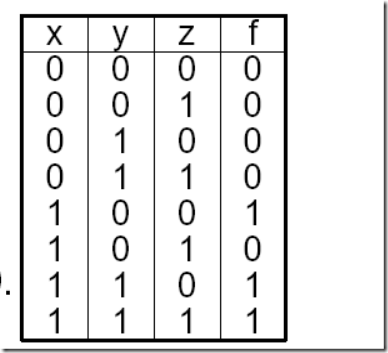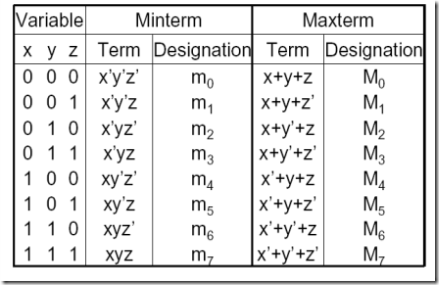### Digital Logic Circuits–Boolean Function

Boolean Function

A Boolean function is an algebraic expression consists of binary variables, the

constants 0 & 1, and the Boolean operators.For a set of given values of the variables,

the function is evaluated to either 0 or 1

e.g. f = x • y + x • z’

The Boolean function f has 3 binaryvariables x, y and z

The function is 1 if x and y are both 1 or if x is 1 and z is 0. Otherwise, f = 0

Operator Precedence

The operator precedence is:

1. Parentheses

2. NOT

3. AND

4. OR

e.g. f = x • y + x • z’

Precedence: z’, x • y, x • z’, x • y + x • z’

e.g. f = (a +b) • (c+d’)

Precedence: a+b, d’, c+d’, (a +b) • (c+d’)

The parentheses precedence is the same as in normal algebra

Boolean Function Truth Table

Boolean function can be represented by truth table as well.If the function has n variables, its truth table will have 2n rows

e.g. f = x • y + x • z’

f has 3 variables so 23 combinations

f is 1 when the expression is evaluated to 1 otherwise it is 0.Minterm

In a Boolean function, a binary variable (x) may appear either in its normal form (x) or in its complement form (x’).Consider 2 binary variables x and y and an AND operation, there are 4 and only 4 possible combinations: x’•y’, x’•y, x•y’ & x•y

Each of the 4 product terms is called a MINTERM or STANDARD PRODUCT

By definition, a Minterm is a product which consists of all the variables in the normal form or the complement form but NOT BOTH.

e.g. for a function with 2 variables x and y:

x•y’ is a minterm but x’ is NOT a minterm

e.g. for a function with 3 variables x, y andz:

x’yz’ is a minterm but xy’ is NOT a minterm

Maxterm

Consider 2 binary variables x and y and an OR operation, there are 4 and only 4

possible combinations: x’+y’, x’+y, x+y’, x+y.Each of the 4 sum terms is called a

MAXTERM or STANDARD SUM.By definition, a Maxterm is a sum in which each variable appears once and only once either in its normal form or its complement

form but NOT BOTH.

Minterms and Maxterms for 3 VariablesMinterm Boolean Expression

Boolean functions can be expressed with minterms,

e.g.f1(x,y,z) = m1 + m4 + m6 = Σm(1, 4, 6)

f2(x,y,z) = m2 + m4 + m6+ m7

= Σm(2, 4, 6, 7)

Maxterm Boolean Expression

Boolean functions can also be expressed with maxterms,

e.g.f1’ = x’y’z’+x’yz’+x’yz+xy’z+xyz

f1 = (x’y’z’+x’yz’+x’yz+xy’z+xyz)’

= (x+y+z)(x+y’+z)(x+y’+z’)(x’+y+z’)(x’+y’+z’)

= M0•M2•M3•M5•M7

= Π M(0, 2, 3, 5, 7)

f2 = M0•M1•M3•M5

= Π M(0, 1, 3, 5)

Literal

A Literal is a variable in a product or sum term

xy’ is a 2-literal product term

x’yz has 3 literals

x’ + xy’ + x’yz is an expression of sum of products with 3 product terms.The 3 product terms have 1, 2 and 3 literals respectively

x’(x+y’)(x’+y+z) is an expression of product of sums.The 3 sum terms have 1, 2 and 3 literals

Express Boolean Functions in Minterms

If product terms in a Boolean function are not minterms, they can be converted to minterms

e.g. f(a,b,c) = a’ + bc’ + ab’c

Function f has 3 variables, therefore, each minterm must have 3 literals

Neither a’ nor bc’ are minterms.They can be converted to minterm.ab’c is a minterm

Conversion to Minterms

e.g. f(a,b,c) = a’ + bc’ + ab’c

To convert a’ to a minterm, the 2 variables (b, c) must be added, without changing its

functionality .Since a’=a’•1 & 1 = b+b’, a’= a’(b + b’) = a’b + a’b’

Similarly, a’b = a’b(c + c’) = a’bc + a’bc’ and a’b’ = a’b’(c+c’) = a’b’c + a’b’c’

bc’ = bc’(a+a’) = abc’ + a’bc’

f = a’bc+a’bc’+a’b’c+a’b’c’+abc’+a’bc’+ab’c

Express Boolean Functions in Maxterms

By using the Distribution Law: x+yz = (x+y)(x+z), a Boolean function can

be converted to an expression in product of maxterms

e.g. f(a,b,c) = a’+bc’

= (a’+b)(a’+c’) {not maxterms}

= (a’+b+cc’)(a’+c’+bb’) {cc’=0}

= (a’+b+c)(a’+b+c’)(a’+c’+b)(a’+c’+b’)

= (a’+b+c)(a’+b+c’)(a’+c’+b’)

Boolean Function Manipulation

Boolean functions can be manipulated with Boolean algebra.Manipulation can transform logic expressions, but still keep the same logic functionality.Manipulation can reduce the complexity, hence, easier to be implemented in hardware, i.e. fewer logic gates

Boolean Function Manipulation Example

f = xy’ + xyz + x’z

= x(y’ + yz) + x’z {common factor}

= x[(y’+y)(y’+z)] + x’z {Distribution law}

= x(y’+z) + x’z {y’ + y = 1}

= xy’ + xz + x’z {Distribution law}

= xy’ + (x + x’)z {common factor}

= xy’ + z {x + x’ = 1}

Simplify f1=abc+a’b+abc’ and f2=(a+b)’(a’+b’) to the minimum literals

f1 = abc+a’b+abc’ = ab(c+c’) + a’b = ab + a’b = (a+a’)b = b

f2 =(a+b)’(a’+b’) = a’b’(a’+b’) {DeMorgan}

= a’b’a’+a’b’b’

= a’b’ + a’b’ = a’b’